NEET  >  Test: Combination of Resistors: Series & Parallel (NCERT)

# Test: Combination of Resistors: Series & Parallel (NCERT)

Test Description

## 20 Questions MCQ Test Physics Class 12 | Test: Combination of Resistors: Series & Parallel (NCERT)

Test: Combination of Resistors: Series & Parallel (NCERT) for NEET 2022 is part of Physics Class 12 preparation. The Test: Combination of Resistors: Series & Parallel (NCERT) questions and answers have been prepared according to the NEET exam syllabus.The Test: Combination of Resistors: Series & Parallel (NCERT) MCQs are made for NEET 2022 Exam. Find important definitions, questions, notes, meanings, examples, exercises, MCQs and online tests for Test: Combination of Resistors: Series & Parallel (NCERT) below.
Solutions of Test: Combination of Resistors: Series & Parallel (NCERT) questions in English are available as part of our Physics Class 12 for NEET & Test: Combination of Resistors: Series & Parallel (NCERT) solutions in Hindi for Physics Class 12 course. Download more important topics, notes, lectures and mock test series for NEET Exam by signing up for free. Attempt Test: Combination of Resistors: Series & Parallel (NCERT) | 20 questions in 20 minutes | Mock test for NEET preparation | Free important questions MCQ to study Physics Class 12 for NEET Exam | Download free PDF with solutions
 1 Crore+ students have signed up on EduRev. Have you?
Test: Combination of Resistors: Series & Parallel (NCERT) - Question 1

### In the series combination of two or more than two resistances

Detailed Solution for Test: Combination of Resistors: Series & Parallel (NCERT) - Question 1

In series combination, current across its circuit components is always constant and in parallel combination the voltage across the circuit components is constant.

Test: Combination of Resistors: Series & Parallel (NCERT) - Question 2

### Match the Column I with Column II.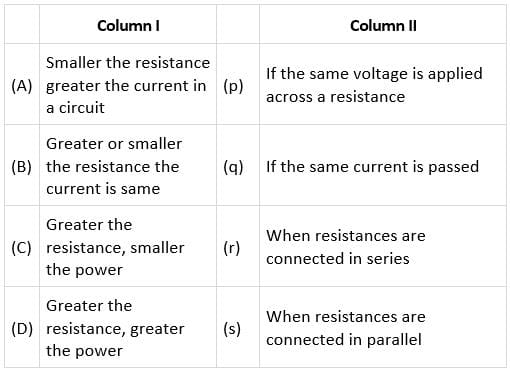Detailed Solution for Test: Combination of Resistors: Series & Parallel (NCERT) - Question 2
• In a parallel combination of resistances, the voltage drop across all the resistors is the same and therefore, the current across the resistances depends on the value of resistance. If the resistance is small, the current flowing through it will be more.
• In a series combination of the resistances, the current through all the resistors is constant whereas the voltage differs for the resistors.
• Similarly, if the same voltage is applied across all the resistors, the power is inversely proportional to the resistance. So, greater the resistance, smaller will be the power and if the current is the same in the circuit, the power will be greater for the higher resistance.
So, A → s
B → r
C → p
D → q
Test: Combination of Resistors: Series & Parallel (NCERT) - Question 3

### Combine three resistors 5Ω, 4.5Ω and 3Ω in such a way that the total resistance of this combination is maximum

Detailed Solution for Test: Combination of Resistors: Series & Parallel (NCERT) - Question 3

For maximum equivalent or total resistance of the resistors must be combined in series.
Req = R+ R2 = R3 = 5 + 4.5 + 3
= 12.5Ω

Test: Combination of Resistors: Series & Parallel (NCERT) - Question 4

The total resistance in the parallel combination of three resistances 9Ω, 7Ω and 5Ω

Detailed Solution for Test: Combination of Resistors: Series & Parallel (NCERT) - Question 4

In the parallel combination of three resistance's resistance is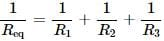or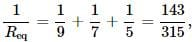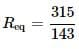= 2.02Ω

Test: Combination of Resistors: Series & Parallel (NCERT) - Question 5

Equivalent resistance (in ohm) of the given network is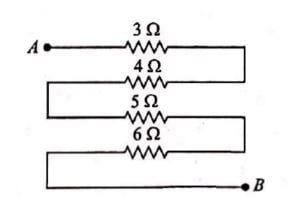Detailed Solution for Test: Combination of Resistors: Series & Parallel (NCERT) - Question 5

Between points A and B all resistances are combined in series
∴ Req = 3Ω + 4Ω + 5Ω + 6Ω
= 18Ω
Between points A and B all resistances are combined in series
∴ Req = 3Ω + 4Ω + 5Ω + 6Ω
= 18Ω

Test: Combination of Resistors: Series & Parallel (NCERT) - Question 6

Which arrangement of 3Ω resistors will give a total resistance of 7Ω?

Detailed Solution for Test: Combination of Resistors: Series & Parallel (NCERT) - Question 6

Three resistors of 3Ω each in parallel will give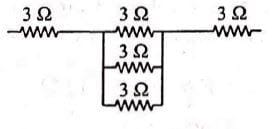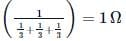Req = 3 + 1 + 3 = 7Ω.

Test: Combination of Resistors: Series & Parallel (NCERT) - Question 7

The equivalent resistance of series combination of four equal resistors is S. If they are joined in parallel, the total resistance is P. The relation between S and P is given by S = nP. Then the minimum possible value of n is

Detailed Solution for Test: Combination of Resistors: Series & Parallel (NCERT) - Question 7

Let Resistance of each resistor is = R
In series connection:
Req1 = 4R = S
R = S/4 → (1)
In parallel connection
Req2 = R4 = P
R = 4/P → (2)
Equating (1) and (2)
S/4 = 4P
S = 16P
n = 16

Test: Combination of Resistors: Series & Parallel (NCERT) - Question 8

Five equal resistances of 10Ω are connected between A and B as shown in figure. The resultant resistance is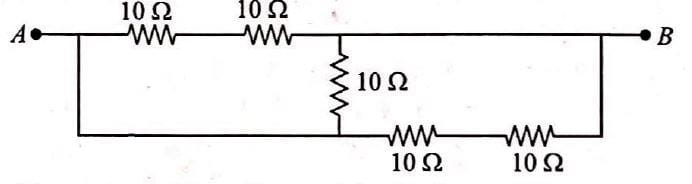Detailed Solution for Test: Combination of Resistors: Series & Parallel (NCERT) - Question 8

According to the given circuit 10Ω and 10Ω resistances are connected in series.
∴ R′ = 10 + 10 = 20Ω
Again 10Ω and 10Ω resistances are connected in series
∴ R′′ = 10 + 10 = 20Ω
R′, R′′ and 10Ω all connected in parallel than
∴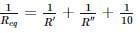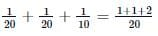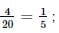Req = 5Ω

Test: Combination of Resistors: Series & Parallel (NCERT) - Question 9

The correct combination of three resistances 1Ω, 2Ω and 3Ω to get equivalent resistance 11/5Ω is

Detailed Solution for Test: Combination of Resistors: Series & Parallel (NCERT) - Question 9

According to option (d) 2Ωand3Ω are combined in a parallel combination. Hence equivalent resistance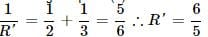R' combined in series to 1Ω
∴ Req =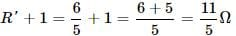Hence, the combination scheme in option (d) is correct.

Test: Combination of Resistors: Series & Parallel (NCERT) - Question 10

Equivalent resistance of the given network between points A and B is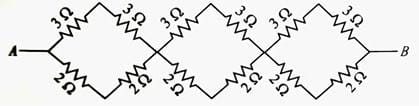Detailed Solution for Test: Combination of Resistors: Series & Parallel (NCERT) - Question 10

In each segment of the combination 3Ω and 2Ω resistance are connected in series separately.
∴ R' = +3 = 6Ω and R'' = 2 + 2 = 4Ω
R' and R'' are connected in parallel
∴ For first segment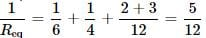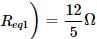Similarly for second and third segment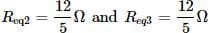Now segment is connected in series then the total resistance of combination is
Req = Req1 + Req2 + Req3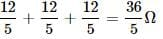Test: Combination of Resistors: Series & Parallel (NCERT) - Question 11

n resistors each of resistance R first combine to give maximum effective resistgance and then combine to give minimum. The ratio of the maximum resistance is

Detailed Solution for Test: Combination of Resistors: Series & Parallel (NCERT) - Question 11

To get maximum equivalent resistance all resistances must be connected in series
∴ (Req)max = R + R + R...ntimes = nR
To get minimum equivalent resistance all resistances myst be connected in parallel.
∴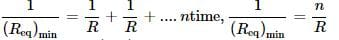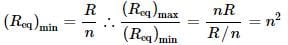Test: Combination of Resistors: Series & Parallel (NCERT) - Question 12

The equivalent resistance between A and B for . the circuit shown in this figure is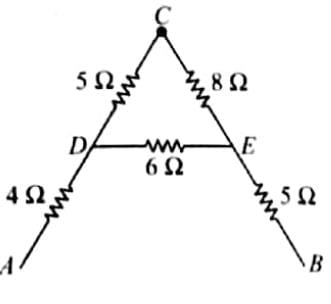Detailed Solution for Test: Combination of Resistors: Series & Parallel (NCERT) - Question 12

For a equivalent resistance betweeen A and B.
5Ω and 8Ω resistance are connected in series. R' their equivalent resistance is parallel to 6Ω
∴ R = 5 + 8 = 13Ω and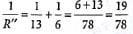R" = 78/19
Now 4Ω, R" an d 5Ω resistances are connected in series equivalent resistance between ,A and B
∴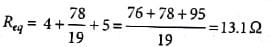Test: Combination of Resistors: Series & Parallel (NCERT) - Question 13

A copper cylindrical tube has inner radius a and outer radius b. The resistivity is ρ. The resistance of the cylinder between the two ends is

Detailed Solution for Test: Combination of Resistors: Series & Parallel (NCERT) - Question 13

If one had considered a solid cylinder of radius b, one can suppose that it is made of two concentric cylinders of radius a and the outer part, joined along the length concentrically one inside the other.
If Ia and Ix are the currents flowing through the inner and outer cylinders
∵ Itotal = Ib = Ia + Ix
⇒ VRb = V/Ra + V/Rx
where Rb is the total resistance and Rx is the resistance of the tubular part.
∴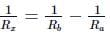But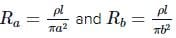∴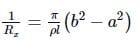∴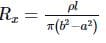Test: Combination of Resistors: Series & Parallel (NCERT) - Question 14

A wire of resistance 12 ohms per meter is bent to form a complete circle of radius 10cm. The resistance between its two diametrically opposite points, A and B as shown in the figure is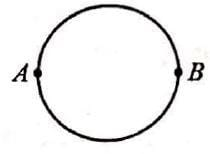Detailed Solution for Test: Combination of Resistors: Series & Parallel (NCERT) - Question 14

Wire of length 2π x 0.1 m of 12Ωm-1 is bent to a circle.Resistance of each part = 12 x π x 0.1
= 1.2π Ω
Total resistance = 0.6π Ω

Test: Combination of Resistors: Series & Parallel (NCERT) - Question 15

A  and B are two points on a uniform ring of resistance 15Ω. The ∠AOB = 45. The equivalent resistance between A and B is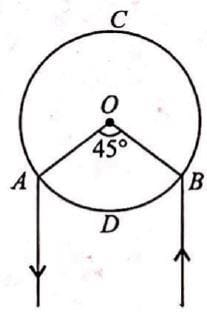Detailed Solution for Test: Combination of Resistors: Series & Parallel (NCERT) - Question 15

Resistance per unit length of ring,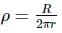Length of sections ADB and ACB are rθ and r(2π − θ)
∴ Resistance of section ADB,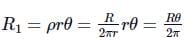and resistance of section ACB,
R2 = ρr(2π − θ)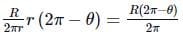Now, R and R2 are connected in parallel between A and B then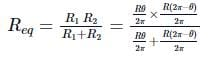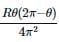Putting θ = 45∘ = π/4 rad and R = 15Ω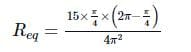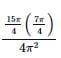= 1.64Ω

Test: Combination of Resistors: Series & Parallel (NCERT) - Question 16

Two metal wires of identical dimensions are connected in series. If σ1 and σ2 are the conductivities of the metal wires respectively, the effective conductivity of the combination is

Detailed Solution for Test: Combination of Resistors: Series & Parallel (NCERT) - Question 16

Resistance of a wire in terms of connectivity (σ) is given by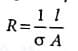where l is the length and A is area of cross section of wire respectively.
∴ Rs = R1 + R2
⇒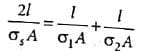where σs is the effective conductivity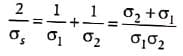σs = 1σ/ σ + σ2

Test: Combination of Resistors: Series & Parallel (NCERT) - Question 17

Three resistors 2 Ω, 4 Ω and 5 Ω are combined in parallel. This combination is connected to a battery of emf 20 V and negligible internal resistance, the total current drawn from the battery is

Detailed Solution for Test: Combination of Resistors: Series & Parallel (NCERT) - Question 17

Potential of 20V20V will be same across each resistance current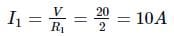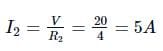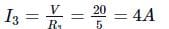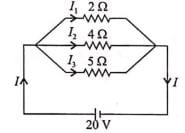∴ Total current drawn from circuit

I = I1 + I2 + I3

= 10 + 5 + 4 = 19A

Test: Combination of Resistors: Series & Parallel (NCERT) - Question 18

Three resistors of resistances 3Ω,4Ω and 5Ω are combined in parallel. This combination is connected to a battery of emf 12V and negligible internal resistance, current through each resistor in ampere is?

Detailed Solution for Test: Combination of Resistors: Series & Parallel (NCERT) - Question 18

Since the voltage across the circuit is constant Then current through 3Ω resistor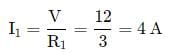The current through 4 £2 resistor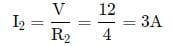and the current through 5 £2 resistor,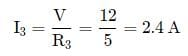Test: Combination of Resistors: Series & Parallel (NCERT) - Question 19

The reading of ammeter shown in figure is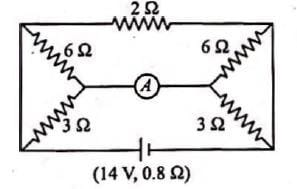Detailed Solution for Test: Combination of Resistors: Series & Parallel (NCERT) - Question 19

The equivalent circuit of the given circuit will be reduced to as shown in figure.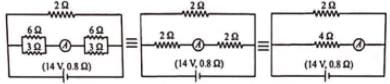Total resistance of the circuit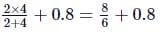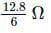Total current in the circuit =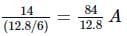Reading of ammeter =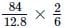= 2.18A

Test: Combination of Resistors: Series & Parallel (NCERT) - Question 20

Three resistances 2Ω, 4Ω, 5Ω are combined in series and this combination is connected to a battery of 12 V emf and negligible internal resistance. The potential drop across these resistances are

Detailed Solution for Test: Combination of Resistors: Series & Parallel (NCERT) - Question 20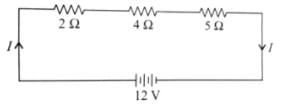Let current in the circuit is I. Then total resistance in the circuit.
R = R1 + R2 + R3 = 2 + 4 + 5 = 11Ω
∴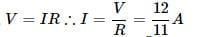The potential drop across 2Ω resistance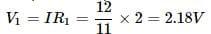The potential drop across 4Ω resistance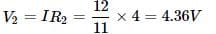The potential drop across 5Ω resistance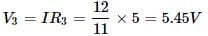Hence(V1, V2, V3) = (2.18, 4.36, 5.45)V

## Physics Class 12

157 videos|452 docs|213 tests
 Use Code STAYHOME200 and get INR 200 additional OFF Use Coupon Code
Information about Test: Combination of Resistors: Series & Parallel (NCERT) Page
In this test you can find the Exam questions for Test: Combination of Resistors: Series & Parallel (NCERT) solved & explained in the simplest way possible. Besides giving Questions and answers for Test: Combination of Resistors: Series & Parallel (NCERT), EduRev gives you an ample number of Online tests for practice

## Physics Class 12

157 videos|452 docs|213 tests

### How to Prepare for NEET

Read our guide to prepare for NEET which is created by Toppers & the best Teachers